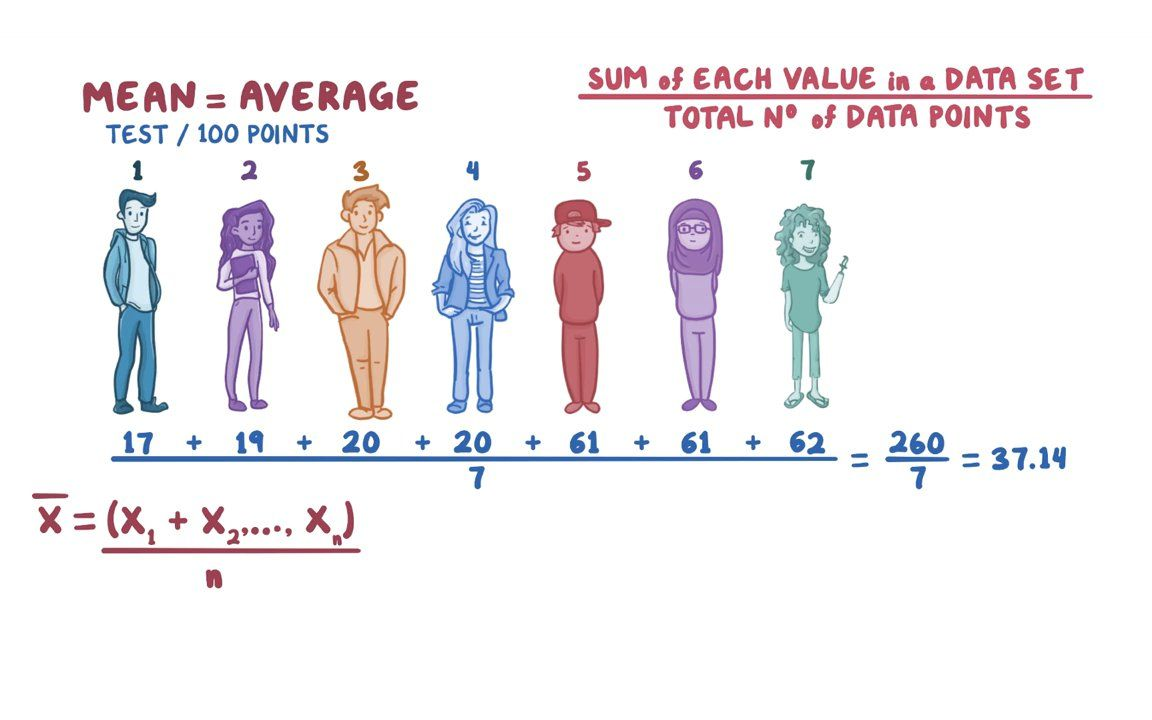[Image_Link]https://www.facebook.com/tr?id=824012811003867&ev=PageView&noscript=1 />

# Mean, median, and mode00:00 / 00:00

## Mean, median, and mode

Biostatistics

### Assessments

Mean, median, and mode

0 / 13 complete

0 / 2 complete

0 / 2 complete

of complete

of complete

### USMLE® Step 2 style questions USMLE

of complete

An investigator is evaluating how many books high school students read each year. Ten students are asked and answered as follows: 4, 3, 7, 8, 6, 13, 2, 10, 12, 16. What is the median number of books read by high school students included in this study?

### Transcript

#### Contributors

In statistics, it’s often helpful to know the central point of a set of data, because it gives a pretty good idea about the whole data set. It’s like a one number summary of the data.

What’s the number of words on a page in a book? About 250. Of course it depends on the book, but that’s the one number summary.

The mean, median and mode are the most commonly used ways to measure this central point.

You can calculate the mean by adding up each value in a data set and then dividing by the total number of data points.

Let’s look at an example. Let’s say 7 students took a test on biostatistics and out of 100 possible points, one student got 17, another got 19, two got 20, two more got 61 and the last student got 62.

The mean score would be the total number of points they all got added up together divided by the number of students, which is 7.

So that’s: 17+19+20+20+61+61+62 = 260 = 37.14

To show this as a formula, we can say that the mean, written as X with a bar over it, is the total sum of the individual data points X1, X2, ......., Xn, divided by n, which is the number of data points.

A mean test score of 37.14, quickly tells us that overall, these students didn’t do well on this test.

But, the problem with the mean, is that it can be influenced by an extreme value called an outlier.

Let’s say that another student comes along and get a perfect 100 out of 100 on the test.

That means that the average is now: 17+19+20+20+61+61+62+100 = 360 divided by 8, which is 45.

This one number summary isn’t a very good summary because the only reason that it is so high is due to this one high-scoring student.

In this case, 100 is an outlier, and any data set with an outlier is called skewed data.

To calculate the central point when there may be outliers, you can use the median.

### Summary

Mean, median, and mode are all measures of "central tendency" - they tell something about the distribution of data points in a set.

The mean is simply the average of all the data points in a set. The median is the middle value in a set (if you ordered the values from smallest to largest, the median would be the value that falls in the middle). And mode is just the value that appears most often in a set.

All three measures are useful for different things. The mean is good for finding out what an average value is, the median is good for identifying outliers (values that are far from the rest of the data), and the mode is good for getting a sense of how clustered or spread out the values in a set are.

Copyright © 2023 Elsevier, its licensors, and contributors. All rights are reserved, including those for text and data mining, AI training, and similar technologies.

Cookies are used by this site.

USMLE® is a joint program of the Federation of State Medical Boards (FSMB) and the National Board of Medical Examiners (NBME). COMLEX-USA® is a registered trademark of The National Board of Osteopathic Medical Examiners, Inc. NCLEX-RN® is a registered trademark of the National Council of State Boards of Nursing, Inc. Test names and other trademarks are the property of the respective trademark holders. None of the trademark holders are endorsed by nor affiliated with Osmosis or this website.